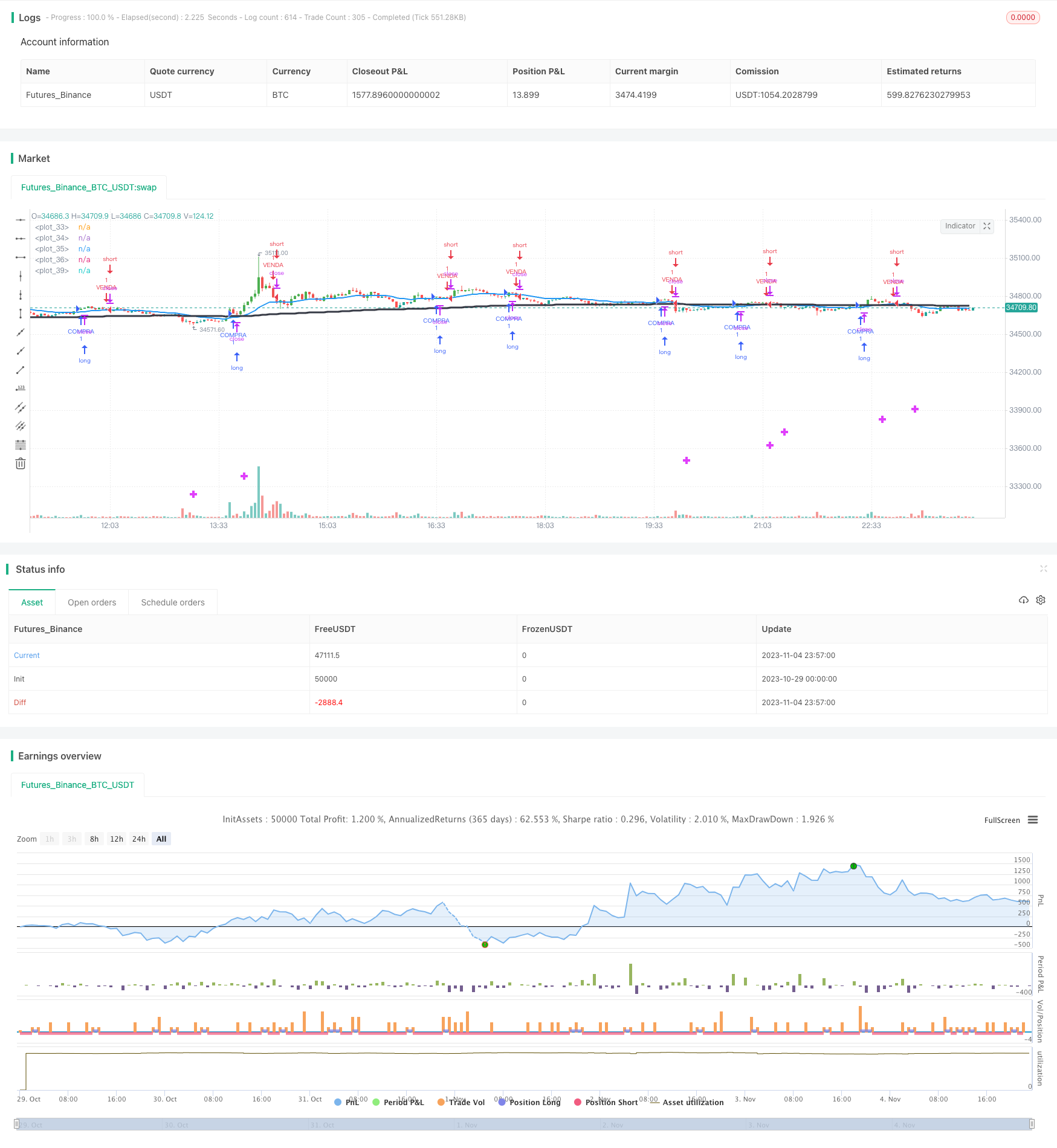# Dual Moving Average Crossover Trend Trading Strategy

Author: ChaoZhang, Date: 2023-11-06 10:27:00
Tags:## Overview

This strategy determines market trends by calculating the crossover situations between the 9-day moving average (MA), 20-day MA and 200-day MA. It combines the classic idea of dual MA crossover with the 200-day MA that gauges the long-term trend. This is a relatively stable and reliable trend trading strategy.

## Strategy Logic

This strategy mainly judges price trends by calculating the relationships between the 9-day MA, 20-day MA and 200-day MA.

Firstly, it calculates the 9-day MA and 20-day MA. If the 9-day MA crosses above the 20-day MA, it is a buy signal. If the 9-day MA crosses below the 20-day MA, it is a sell signal. This is the most basic judgment rule of dual MA crossover.

Secondly, it calculates the 200-day MA as an indicator for judging long-term trends. If the 20-day MA crosses above the 200-day MA, it signals a long-term bullish view. If the 20-day MA crosses below the 200-day MA, it signals a long-term bearish view.

Finally, it combines the relationships between the 9-day MA, 20-day MA and 200-day MA to determine specific entry and exit points. Only when the 9-day MA and 20-day MA cross over together in the same direction will actual trading signals be generated.

By calculating the crossover situations between multiple MAs, this strategy makes full use of the trend tracking capability of MAs to effectively determine short-term and long-term price movements, thereby guiding buy and sell operations.

1. Using dual MA crossover can effectively capture mid-short term price trends and generate profits.

2. Adding 200-day MA judgment avoids going long during long-term downtrends, reducing losses.

3. Combining multiple MA relationships makes the signals more reliable and avoids ineffective trades.

4. MA crossover signals are clear and easy to judge, suitable for manual trading practice.

5. The simple and clean code is easy to understand and implement, good for quant trading beginners.

6. Flexible to optimize, like adjusting MA periods or adding other indicators.

## Risk Analysis

1. MA strategies are sensitive to parameter tuning, different MA periods can produce very different results.

2. Dual MA crossover only judges mid-short term trends, may miss longer-term big trends.

3. Crossover signals may lag and cannot completely avoid losing trades.

4. Frequent trading increases commission and slippage costs, reducing actual profit potential.

5. The overly simple code may underperform in live trading, requiring optimization.

## Optimization Directions

1. Test different MA period combinations to find the optimal parameters.

2. Add stop loss strategies to strictly control per trade loss amount.

3. Consider position sizing according to changing market conditions.

4. Optimize entry signals, such as adding Momentum confirmation.

5. Optimize exits by setting reasonable take profit levels.

6. Add more indicators to judge trends and pullback probabilities.

## Conclusion

This strategy combines the classic ideas of dual MA crossover and long-term MA trend judgment to guide trading decisions using MA trend-following characteristics. It has simple logic and is easy to understand and implement, good for quant trading beginners. However, it is parameter sensitive and has lagging issues that require further optimization and improvement. Overall, this strategy provides a basic framework that can be extended upon to develop more powerful trading systems. Investors can choose suitable elements to add and continuously optimize the strategy based on their needs, in order to achieve long-term excess returns in quantitative trading.

```/*backtest
start: 2023-10-29 00:00:00
end: 2023-11-05 00:00:00
period: 3m
basePeriod: 1m
exchanges: [{"eid":"Futures_Binance","currency":"BTC_USDT"}]
*/

//@version=1
strategy("Dieyson Swingtrade EMA 20+200 and bar & line color", overlay=true)

//bar color rules
Dgbar = close>close and ema(close,20)>ema(close,20)
Drbar = close<close and ema(close,20)<ema(close,20)

//Barcolors
barcolor(Dgbar ? green : na)
barcolor(Drbar ? red : na)

//MM09 Colorful

MMgreen9 = ema(close,9)>ema(close,9) and ema(close,20)>ema(close,20)
MMred9 = ema(close,9)<ema(close,9) and ema(close,9)<ema(close,9)
col8 = (MMgreen9 ? color(green,0) : na)
col28 = (MMred9 ? color(red,0) : na)
col38 = (not MMgreen9 and not MMred9 ? color(black,0) : na)

//plot(ema(close,9), color=col8, style=line, linewidth=1)
//plot(ema(close,9), color=col28, style=line, linewidth=1)
//plot(ema(close,9), color=col38, style=line, linewidth=1)

//MM20 Colorful

MMgreen = ema(close,20)>ema(close,20)
MMred = ema(close,20)<ema(close,20)
col = (MMgreen ? color(green,0) : na)
col2 = (MMred ? color(red,0) : na)
col3 = (not MMgreen and not MMred ? color(yellow,0) : na)
col4 = color(black,0)
plot(ema(close,20), color=col, style=line, linewidth=2)
plot(ema(close,20), color=col2, style=line, linewidth=2)
plot(ema(close,20), color=col3, style=line, linewidth=2)
plot(ema(close,200), color=col4, style=line, linewidth=3)
//plot(vwap(15), color(white,0), style=line, linewidth=3)
//plot(cross(ema(close,9), ema(close,20)) ? ema(close,9) : na, style = cross,color=fuchsia, transp=0, linewidth = 4)
plot(cross(ema(close,20), ema(close,200)) ? ema(close,20) : na, style = cross,color=fuchsia, transp=0, linewidth = 4)

c = crossover(ema(close,9), ema(close,20)) and ema(close,9) > ema(close,20)
// c = crossover(close, ema (close,9) and ema(close,9) > ema(close,9))
v = crossunder(close, ema (close,9))

strategy.entry("COMPRA", strategy.long,when=c)
strategy.entry("VENDA", strategy.short,when=v)

```

More